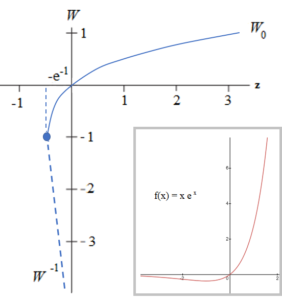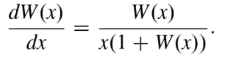# Lambert W function (Omega Function): Simple Definition

Simply put, the Lambert W function is the inverse function of f(x) = xex.Graph of the Real-valued branch of the Lambert W function (blue line), and the function f(x) = xex (inset, red).

The graph above shows the real-valued branches for Lambert’s W. The graph is split into two parts (indicated by the blue dot at (-e-1, -1)), each of which share a tangent line.

• The blue line (W0) is the inverse of the function f(x) = xex with the function restricted to y-values ≥ -1. This is sometimes called the main branch because it goes through the origin.
• The rest of the function(W-1) curves in on itself (shown by the dashed blue line), creating multiple outputs for the same x-value. This part of the function violates the “each input maps to a single output” definition of a function.

The “piecewise” nature of the function is part of what makes Lambert’s W unusual and challenging to deal with.

Lambert’s W function, which can be real-valued or complex-valued, is a non elementary function used extensively in practically every scientific field including chemistry, computer science, mechanical engineering and physics. Its main purpose is to express solutions for equations where an unknown variable is present in an exponent and in another part of the equation.

## Mathematical Definition

The Lambert W function can be defined mathematically as a solution to the following equation (Shynk, 2016):

x = w ew

The set of solutions are called branches. The domain of the function is (-e-1, ∞) and the range is (-∞, ∞).

## A Challenging Function

Although the function is in widespread use, one of the major challenges with the Lambert W function is the lack of standard name and notation (Corless et al., 1996). As well as the fairly straightforward definition above, the function is defined in many different ways, including:

Another challenge of using the function is that the variable “w” in the Lambert W function cannot be expressed as a function of x, even by taking the logarithm of both sides.

Finally, this unusual function has no symmetry. Therefore, it can’t be classified as either an odd or even function.

## First Order Derivative Lambert Function

The first order Lambert W function is found by differentiating the functional equation defined above. When you do that, you get:## References

Corless, R. et al. (1996). On the Lambert W function. Advances in Computational Mathematics 5(1), 329-59.
Desmos Graphing Calculator.
Dobrushkin, V. MATHEMATICA TUTORIAL for the Second Course. Part I: Basic Matrix Operations.
Joyce, D. (2015). What is a simplified explanation of the Lambert W function?
Retrieved December 7, 2019 from: https://www.quora.com/What-is-a-simplified-explanation-of-the-Lambert-W-function
Pardalos, P. & Themistocles, R. (2016). Contributions in Mathematics and Engineering: In Honor of Constantin Carathéodory. Springer.
Sanguin, C. (2013). Computer Aided Assessment of Mathematics. OUP Oxford.
Shynk, J. (2016). Mathematical Foundations for Linear Circuits and Systems in Engineering. John Wiley & Sons.
Pardalos, P. & Themistocles, R. (2016). Contributions in Mathematics and Engineering: In Honor of Constantin Carathéodory. Springer.
Sanguin, C. (2013). Computer Aided Assessment of Mathematics. OUP Oxford.
Shynk, J. (2016). Mathematical Foundations for Linear Circuits and Systems in Engineering. John Wiley & Sons.

CITE THIS AS:
Stephanie Glen. "Lambert W function (Omega Function): Simple Definition" From StatisticsHowTo.com: Elementary Statistics for the rest of us! https://www.statisticshowto.com/lambert-w-function-omega/